# Alternating Offers Protocol

(Rubinstein)

[Contributed by Paolo Ballarini, Michael Fisher & Michael Wooldridge]

### Contents

Related publications: [BFW06]

### Introduction

This case study is about the analysis of a Negotiation Framework known as Rubinstein's Alternating Offers Protocol [OR90]. In such a framework two agents, the Buyer (B) and the Seller (S), bargain over an item. Players alternatively take it in turns to make an action which can be either (i) throwing a proposal (offer), or (ii) accepting the most recent proposal. In theory both players can keep Rejecting offers so that an agreement may never be reached (in that case we talk about disagreement or conflict deal). However a player's utility depends on the value at which an agreement is reached (as well as on the time at which it is reached), hence disagreement is the worst possible outcome for both players and it is ruled out. The following assumptions are considered:

• Disagreement is the worst outcome: players prefer any outcome at least as much as disagreement
• Players seek to maximise utility: players really do prefer to get larger utility values.
• Time is valuable: for any outcome x and times t1 and t2 with t1<t2, outcome x at time t1 has a higher utility than outcome x at time t2 for both players.

### Players' strategies (conceder/boulware)

Players' offers are calculated by means of the so-called Negotiation Decision Function (NDF), a function of time that determines a player's strategy. Strategies can be linear or non-linear, the latter being either conceder, if the player is willing to concede a lot in the early phase of negotiation, or boulware if a player is willing to concede considerably only when its time deadline is approaching. The following parameters are relevant for the characterisation of a player strategy.

• T_b (Time-deadline ): player b quits negotiation if an agreement has not been reached within T_b.
• IP_b (Initial Price): player b's first offer.
• RP_b (Reserved Price): the threshold above (below) which player b will certainly reject offer (hence also its maximum/minimum offered value, i.e. player b offers RP_b only at time T_b).

The picture below shows how different buyer's strategies graphically compare.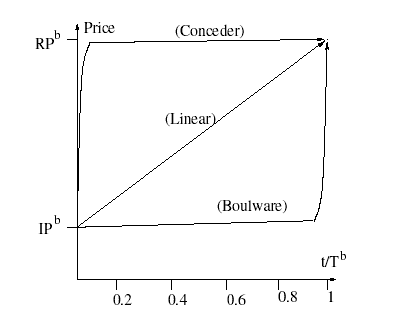### Acceptance probability

The uncertain nature of an offer evaluation outcome is represented by making the decision whether to accept or reject a player's offer a probabilistic one. The will of a player (b) to accept an offer is affected by both the offered value and the player's reserved price (RP_b). The following points are considered for the characterisation of players' acceptance probability (functions S_AP() and B_AP() below):

1. Any offer below (above) the S_RP (B_RP) will be certainly rejected by the Seller (Buyer).
2. The probability of accepting an offer (counter-offer) asymptotically tend to 1 as the offer (counter-offer) increases (decreases).
3. For the sake of symmetry, players should have an equal attitude towards acceptance/rejection of an offer, which is: players are equally likely to accept offers of equal utility (i.e. offers whose distance from their respective RP is the same).

The resulting acceptance probability functions are as follows:

 S_AP(x)    = 0 if x ≤ S_RP 1- S_RP/2 if x > S_RP

 B_AP(x)    = 1 if x ≤ 0 1+ S_RP/(x - (B_RP + S_RP)) if 0 < x < B_RP 0 if x > B_RP

The following picture depicts the graph for functions S_AP, B_AP with S_RP = 1000 and B_RP = 10000. It is straightforward to see that functions S_AP, B_AP as in the above definition actually fulfil the described guidelines.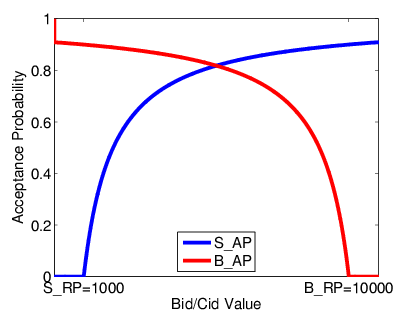### The model

Rubinstein's Negotiation Protocol has been modelled in PRISM as a DTMC consisting of two parallel modules which synchronise on certain actions. The behaviour of each module is based on the following state-chart diagrams:The model is designed to cope with different negotiation strategies (linear, non-linear), the desired strategy being set through model's configuration. The set of configuration parameters is listed below.

• Players' strategy: any possible combination of strategies can be chosen for the two players (e.g. (Boul,Conc) represents a setting such that the Buyer is using a Boulware strategy whereas the Seller a Conceder one). Player b's (either B or S) strategy is set by means of the module's b boolean variable bcon (either Bcon or Scon). A true value for such a variable denotes a conceder strategy, whereas false denotes a boulware one. Linear strategies can be obtained by setting a switch time greater than the time deadline, hence the value given to bcon is irrelevant when the desired strategy is a linear.
• Accepting interval [S_RP,B_RP]: set by means of the 2 (global) constants S_RP and B_RP. The chosen interval affects the acceptance probability functions, hence the probabilistic outcome of negotiation. The interval's halves, [S_RP,(S_RP + B_RP)/2] and [(S_RP + B_RP)/2,B_RP] are classified accordingly to a player's utility. Hence [S_RP,(S_RP + B_RP)/2] is the buyer's higher utility half (whereas [(S_RP + B_RP)/2,B_RP] is the buyer's lower utility half), as any agreement in [S_RP,(S_RP + B_RP)/2] has a higher utility, from the buyer point of view, than an agreement within [(S_RP + B_RP)/2,B_RP]. Conversely, [S_RP,(S_RP + B_RP)/2] and [(S_RP + B_RP)/2,B_RP] are respectively the lower utility and higher utility half from the Seller point of view.
• Strategies gradients: set by means of the (global) constants bCinc, bBinc (for the buyer) and sCdec, sBdec (for the seller). Non-linear strategies are approximated via 2-segments-linear ones, consisting of a conceder segment followed by a boulware one, in a conceder tactic, or vice-versa in a boulware strategy. The gradient of the 2 segments are specified separately for the 2 players by assigning a specific value to these constants.
• Bargaining Time Deadlines: set by means of the (global) constants T_s, T_b. These values determine the players' bargaining deadlines.
• Boulware Strategy Switch Time: set by means of the (global) constants TbB, TsB. In a Boulware strategy, TbB ( TsB) is the time instants at which the buyer (seller) starts conceding.
• Conceder Strategy Switching Factor: set by means of the (global) constants Kb, Ks. In a Conceder strategy the buyer (seller) stops conceding when its next offered value is closer than Kp (Ks) times the conceding gradient from its RP.
• Accepting Interval's Offset: set by means of the (global) constant Offset. This constant helps for minimising the model's complexity. If one wants to study the probabilistic outcome for an acceptance interval equal to, say, [a,b], with 0 < a < b < ∞, and width w = b - a, then in order to get the lowest possible model's complexity the actual interval should be set equal to [1,1 + w] (i.e. S_RP = 1 and B_RP = 1 + w) and Offset = a - 1.

The PRISM source code is given below.

```dtmc

//RESERVED PRICE - ACCEPTING INTERVAL [S_RP,B_RP]
// A player's RP characterises the certainty of refusal for a received proposal.
// any offer above(below) the RP is certainly rejected.
// Buyer's and Seller's RPs give the accepting-interval [S_RP,B_RP]
// We assume S_RP<B_RP
const int B_RP = 1000; // Buyer RESERVED_PRICE
const int S_RP = 1; // seller RESERVED_PRICE

// INITIAL PRICE
const int B_IP = S_RP; // Buyer Initial Price
const int S_IP = B_RP; // Seller Initial Price

// A player (b/s) offers its Reserved Price when it meets its time-deadline (Tb/Ts).
// With a Boulware strategy, TbB(TsB) is the time at which a player starts conceding.
// Note: TbB(TsB) must be less than Tb(Ts).
const int TbB =20; // Buyer start Conceding Time-deadline (for Boulware strat.)
const int Ts = 50; // Seller Time-deadline
const int TsB = 48; // Seller Time-deadline from which stops conceding (for Conceder strat.)

// these values characterise Conceder and Boulware gradients for both players.
const int bCinc = 100;  // Buyer Conceder increment
const int bBinc = 1;   // Buyer Boulware increment
const int sCdec = 100;   // Seller Conceder decrement
const int sBdec = 1;   // Seller Boulware decrement

// SWITCHING FACTOR for Conceder strategy.
// A player stops conceding when its next bid is less than Kb(Ks)*bCinc(sCdec) far
// from the player's Reserved Price.
const int Kb = 1; // Buyer's switching factor
const int Ks = 8; // Seller's switching factor

// ACCEPTING INTERVAL'S OFFSET
// this value allows for considering a shifted   accepting interval [S_RP+Offset,B_RP+Offset]
// while preserving model building's complexity (variables values are minimised
// hence model's solution is simpler).
const Offset = 10000; // Offset for the accepting interval [S_RP+Offset,B_RP+Offset]

b:[0..6] init 0;
bid :[B_IP..B_RP] init B_IP;
tb:[0..Tb] init 0; // bid counter
Bcon : bool init false;
Bstop : bool init false;
Bswitch :bool init false;
Bagreed:bool init false;

// 0-  STRATEGY SWITCHING (pre Bid-Throwing)
[] b=0 & !Bswitch & Bcon & B_RP-bid>Kb*bCinc -> 1 : (b'=1);
[] b=0 & !Bswitch & !Bcon & tb<TbB -> 1 : (b'=1);
[] b=0 & Bswitch -> 1 : (b'=1);
// Switch
[] b=0 & !Bswitch & Bcon & B_RP-bid<=Kb*bCinc
-> 1 : (b'=1) & (Bcon'=false) & (Bswitch'=true); // CONCEDER to BOULWARE
[] b=0 & !Bswitch & !Bcon & tb=TbB
-> 1 : (b'=1) & (Bcon'=true) & (Bswitch'=true); // BOULWARE to CONCEDER

// 1- BID THROWING
[BID] b=1 & Sstop ->1 : (b'=4);
[BID] b=1 & tb=0 & !Sstop -> 1 : (b'=6) & (tb'=tb+1); //starting bid

[BID] b=1 & Bcon & tb>0 & tb<Tb-1 & B_RP-bid>bCinc & !Sstop  -> // CONCEDER
1 : (b'=6) & (bid'=bid+bCinc) & (tb'=tb+1); //Conceding Increment
[BID] b=1 & !Bcon & (tb>0 & tb<Tb-1) & (B_RP-bid)>bBinc & !Sstop -> // BOULWARE
1 : (b'=6) & (bid'=bid+bBinc)& (tb'=tb+1); // Boulware Increment
[BID] b=1 & tb>0 & Bcon & !Sstop & (tb=Tb-1 | (tb<Tb-1 & B_RP-bid<=bCinc)) ->
1 : (b'=6) & (bid'=B_RP) & (tb'=tb+1) & (Bstop'=true); // Last Bid reserved price
[BID] b=1 & tb>0 & !Bcon & !Sstop & (tb=Tb-1 | (tb<Tb-1 & B_RP-bid<=bBinc)) ->
1 : (b'=6) & (bid'=B_RP) & (tb'=tb+1) & (Bstop'=true); // Last Bid reserved price

// 6-  STRATEGY SWITCHING (post Bid-throwing)
[] b=6  & !Bswitch & Bcon & B_RP-bid>Kb*bCinc  -> 1 : (b'=2);
[] b=6  & !Bswitch & !Bcon & tb<TbB -> 1 : (b'=2);
[] b=6  & Bswitch -> 1 : (b'=2);
[] b=6  & !Bswitch & Bcon & B_RP-bid<=Kb*bCinc
-> 1 : (b'=2) & (Bcon'=false) & (Bswitch'=true); // CONCEDER to BOULWARE
[] b=6  & !Bswitch & !Bcon & tb=TbB
-> 1 : (b'=2) & (Bcon'=true) & (Bswitch'=true); // BOULWARE to CONCEDER

// 2- WAITING FOR BID ANALYSIS RESULT
[] b=2 & s=2 -> 1 : (b'=3) & (Bagreed'=true);
// 2- COUNTER-BID RECEIVING
[CBID] b=2 & !Bstop -> 1 : (b'=4);
[CBID] b=2 & Bstop -> 1 : (b'=5);

// 3- AGREEMENT REACHED
[PURCHASE] b=3  -> 1 : (b'=3);

// 4- COUNTER-BID ANALYSIS
// Conceder strategy
[] b=4 & Bcon & tb<Tb-1 & bid+bCinc>=cbid  -> 1 : (b'=3);
[] b=4 & Bcon & tb=Tb-1 & B_RP>=cbid -> 1 : (b'=3);
[] b=4 & Bcon & !Sstop & ((tb<Tb-1 & bid+bCinc<cbid) | (tb=Tb-1 & B_RP<cbid)) ->
max(0,1 + (S_RP+Offset)/(cbid-(B_RP+S_RP+Offset))) : (b'=3)
+ 1-max(0,1 + (S_RP+Offset)/(cbid-(B_RP+S_RP+Offset))) : (b'=0);
[] b=4 & Bcon &  Sstop & ((tb<Tb-1 & bid+bCinc<cbid) | (tb=Tb-1 & B_RP<cbid)) ->
max(0,1 + (S_RP+Offset)/(cbid-(B_RP+S_RP+Offset))) : (b'=3)
+ 1-max(0,1 + (S_RP+Offset)/(cbid-(B_RP+S_RP+Offset))) : (b'=5);
// Boulware strategy
[] b=4 & !Bcon & tb<Tb-1 & bid+bBinc>=cbid -> 1 : (b'=3);
[] b=4 & !Bcon & tb=Tb-1 & B_RP>=cbid -> 1 : (b'=3) ;
[] b=4 & !Bcon & !Sstop & tb<Tb-1 & bid+bBinc<cbid ->
max(0,1 + (S_RP+Offset)/(cbid-(B_RP+S_RP+Offset))) : (b'=3)
+ 1-max(0,1 + (S_RP+Offset)/(cbid-(B_RP+S_RP+Offset))) : (b'=0);
[] b=4 & !Bcon & (Sstop=true) & (tb<Tb-1) & ((bid+bBinc)<cbid)->
max(0,1 + (S_RP+Offset)/(cbid-(B_RP+S_RP+Offset))) : (b'=3)
+ 1-max(0,1 + (S_RP+Offset)/(cbid-(B_RP+S_RP+Offset))) : (b'=5);

// 5- HALTING STATE
[STOP] b=5 -> 1 : (b'=5);

endmodule

// THE SELLER
module Seller

s:[0..7] init 0;
cbid :[S_RP..S_IP] init S_IP;
ts:[0..Ts] init 0;     // cbid counter
Scon: bool init true;
Sstop: bool init false;
Sswitch :bool init false;

//  0- BID RECEIVING
[BID] s=0 & !Bstop & !Sstop -> 1 : (s'=1);
[BID] s=0 & (Bstop | Sstop) -> 1 : (s'=5);
// 1- BID ANALYSIS
[] s=1 & Scon & cbid-sCdec<=bid -> 1 : (s'=2);
[] s=1 & Scon & !Bstop & cbid-sCdec>bid
->  max(0,1-(S_RP+Offset)/(bid+Offset)) :(s'=2)
+ 1-max(0,1-(S_RP+Offset)/(bid+Offset)) :(s'=6);
[] s=1 & Scon & Bstop & cbid-sCdec>bid
->  max(0,1-(S_RP+Offset)/(bid+Offset)) :(s'=2)
+ 1-max(0,1-(S_RP+Offset)/(bid+Offset)) :(s'=5);
[] s=1 & !Scon & ts<Ts-1 & cbid-sBdec<=bid -> 1 : (s'=2);
[] s=1 & !Scon & ts=Ts-1 & cbid-sBdec<=bid -> 1 : (s'=2);
[] s=1 & !Scon & !Bstop & cbid-sBdec>bid
->  max(0,1-(S_RP+Offset)/(bid+Offset)) :(s'=2)
+ 1-max(0,1-(S_RP+Offset)/(bid+Offset)) :(s'=6);
[] s=1 & !Scon & Bstop & cbid-sBdec>bid
->  max(0,1-(S_RP+Offset)/(bid+Offset)) :(s'=2)
+ 1-max(0,1-(S_RP+Offset)/(bid+Offset)) :(s'=5);

// 2- AGREEMENT REACHED
[PURCHASE] s=2 -> 1 : (s'=2);

// 6- STRATEGY SWITCHING (pre CBID-throwing)
[] s=6 & !Sswitch & Scon & cbid-S_RP>Ks*sCdec -> 1 : (s'=3);
[] s=6 & !Sswitch & !Scon & ts<TsB -> 1 : (s'=3);
[] s=6 & Sswitch -> 1 : (s'=3);
[] s=6 & !Sswitch & Scon & cbid-S_RP<=Ks*sCdec ->
1 : (s'=3) & (Scon'=false) & (Sswitch'=true); // CONCEDER to BOULWARE
[] s=6 & !Sswitch & !Scon & ts=TsB ->
1 : (s'=3) & (Scon'=true) & (Sswitch'=true); // BOULWARE to CONCEDER

// 3- COUNTER-BID THROWING
[CBID] s=3 & Bstop ->1 : (s'=5);
[CBID] s=3 & ts=0 & !Bstop -> 1 : (s'=7) & (ts'=ts+1);
[CBID] s=3 & Scon & !Bstop & ts>0 & ts<Ts-1 & cbid-S_RP>sCdec ->
1 : (s'=7) & (cbid'=cbid-sCdec) & (ts'=ts+1);  // if Conceder stay Conceder
[CBID] s=3 & !Scon & !Bstop  & ts>0 & ts<Ts-1 & cbid-S_RP>sBdec ->
1 : (s'=7) & (cbid'=cbid-sBdec) & (ts'=ts+1);  // if Conceder stay Conceder
[CBID] s=3  & ts>0 & Scon & !Bstop & (ts=Ts-1 | (ts<Ts-1 & cbid-S_RP<=sCdec)) ->
1 : (s'=7) & (cbid'=S_RP) & (ts'=ts+1) & (Sstop'=true); // Last Bid reserved price
[CBID] s=3  & ts>0 & !Scon & !Bstop & (ts=Ts-1 | (ts<Ts-1 & cbid-S_RP<=sBdec)) ->
1 : (s'=7) & (cbid'=S_RP) & (ts'=ts+1) & (Sstop'=true); // Last Bid reserved price

// 4- WAITING FOR COUNTER-BID ANALYSIS RESULT
[] s=4 & b=1 -> 1 : (s'=0);
[] s=4 & b=3 -> 1 : (s'=2);

// 5- HALTING STATE
[STOP] s=5 -> 1 : (s'=5);

// 7- STRATEGY SWITCHING (post CBID-throwing)
[] s=7 &  !Sswitch & Scon & cbid-S_RP>Ks*sCdec -> 1 : (s'=4);
[] s=7 &  !Sswitch & !Scon & ts<TsB -> 1 : (s'=4);
[] s=7 &  Sswitch -> 1 : (s'=4);
[] s=7 & !Sswitch & Scon & cbid-S_RP<=Ks*sCdec ->
1 : (s'=4) & (Scon'=false) & (Sswitch'=true); // CONCEDER to BOULWARE
[] s=7 & !Sswitch & !Scon & ts=TsB ->
1 : (s'=4) & (Scon'=true) & (Sswitch'=true); // BOULWARE to CONCEDER

endmodule
```

### Analysis

The probabilistic outcome of the Negotiation process is evaluated by computing the probability that an agreement is reached within the Accepting Interval. In practise this is obtained by model-checking the PCTL formula:

P = ? [ true U s=2 & b=3 & (bid=PVAL | cbid=PVAL)]

which captures all the system's evolutions representing a reached agreement.

The Negotiation probabilistic outcome for a given configuration is calculated through experimentation by varying PVAL ∈ [S_RP, B_RP]. Different negotiation strategies can be compared once results for the corresponding configurations are obtained. In the graph below the probabilistic outcome of bargaining (i.e. the acceptance probability) in the interval [10000,11000], is compared for linear symmetric strategies with different gradients (both players use a linear tactic, but what is changed is the increment/decrement value).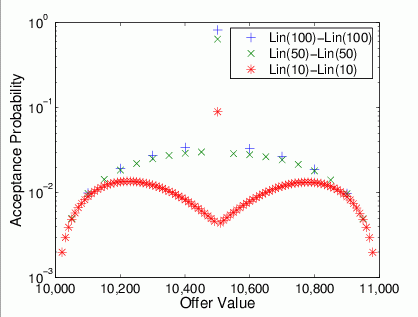#### Linear strategies: Slow is better than Fast.

More interesting, from an analytical point of view, is the cumulative acceptance probability (directly derived from result of experimentation). In the next picture the Cumulative Acceptance Probability is compared for linear strategies with different gradients. One can notice that any curve corresponding to a Seller (slow) decrement equal to 10 (i.e. Lin(10)-Lin(10) or Lin(50)-Lin(10) or Lin(100)-Lin(10)) is "probabilistically" better than any curve corresponding to a Seller (fast) decrement of 100, as it concentrates most of the probability close to the supremum of the Accepting interval, independently of the Buyer's increment. Hence the following general criteria can be devised: from a player's point of view, a slower strategy is to be preferred to a fast one. In other words, for a player p moving the offered value slowly, rather than quickly, towards its RP makes more likely that the outcome of bargaining will fall in its higher utility half of the accepting interval.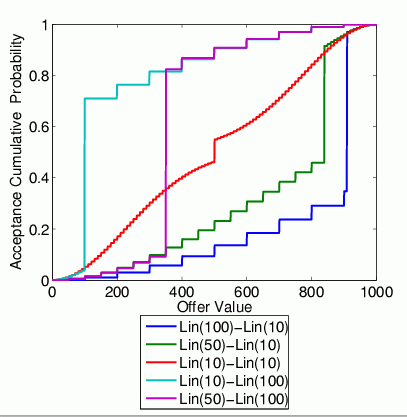#### Non-Linear Strategies: the shorter Conceding time, the better.

In our framework Non-Linear strategies are approximated via 2-segments-linear NDFs. Several relevant parameter must be chosen with a non-linear strategy (either conceder or boulware), like the gradients for both segments and the switch-time, if boulware, or the switching factor, if conceder. The criteria for segments' gradient is as for linear strategies. Hence, in general, slow gradients are to be preferred to fast ones, no matter what non-linear strategies is considered. Furthermore, a comparative analysis of non-linear strategies, has proved that the shorter the conceding segment is, the higher is the probability for the player to get a greater utility out of the negotiation.

This is shown in the figure below where acceptance probability (cumulative) for different combination of Conceder strategies (both player adopting a conceder strategy) are compared by changing the instant of time when the Seller switches from the fast to the slow behaviour. One can observe that the earlier this switching takes place (i.e. TS_sw:4 rather than TS_sw:8 ), the higher is the probability the negotiation outcome will fall close to the interval's supremum, which is good from the Seller point of view.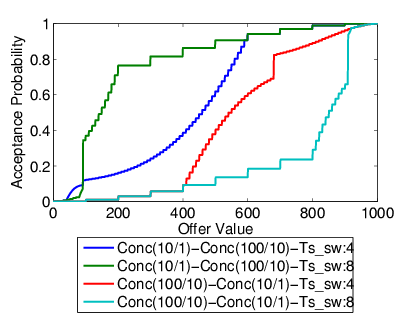The above considerations are valid in general, independently of the chosen non-linear strategy. The graphs depicted in the figure below, compares the acceptance probability (cumulative) for different value of the Seller's switching time (i.e. Ts:9, Ts:6, Ts:2 ) when the Buyer has a boulware tactic, whereas the Seller a conceder one. The curves in there, prove that, independently of the Buyer's tactic, a short conceding time is really in the interest of the Seller.